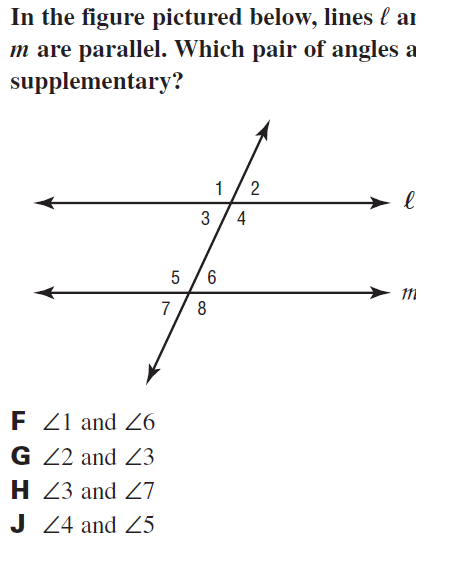Activity 4 parallel lines QUIZ
If <1 = 116 degrees, what is <5? *
2 points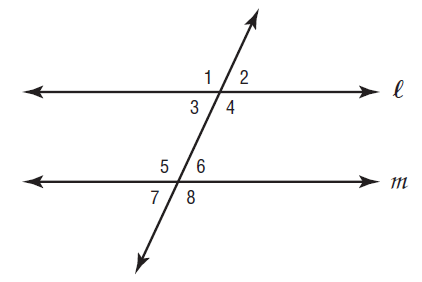Period *
If <2 = 75 and <7 = 65. What is the measure of <13? *
1 point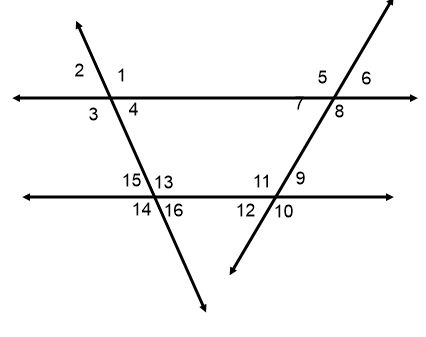solver for x *
1 point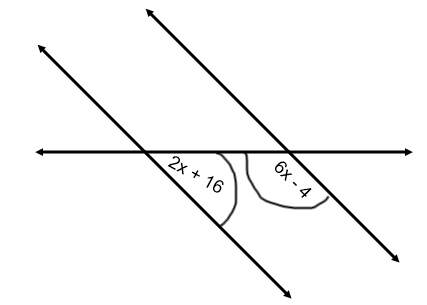If <4 = 106 degrees, what is <6? *
2 pointsACT style question *
1 point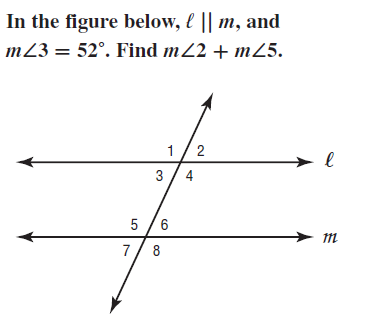Last Name *
Which relationship is always supplementary? *
1 point
Name the relationship *
2 points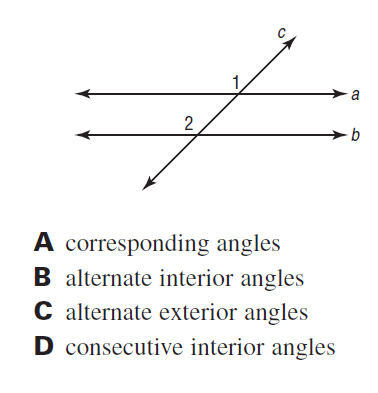Name the relationship between <2 and <7 *
2 pointsIf <5 = 112 degrees and <6 = 3x + 8. Solve for x *
1 point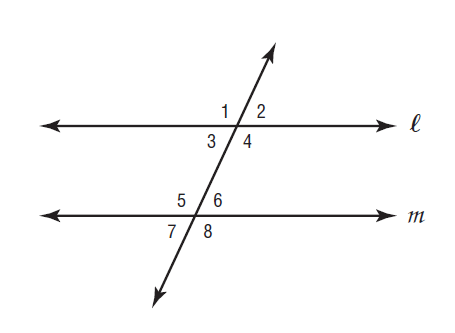ACT style question *
1 point